• 均方误差损失函数（MSE，mean squared error 回归问题解决的是对具体数值的预测，比如房价预测、销量预测等等，解决回归问题的神经网络一般只有一个输出节点，这个节点的输出值就是预测值。本文主要介绍回归问题下的...
均方误差损失函数（MSE，mean squared error)
回归问题解决的是对具体数值的预测，比如房价预测、销量预测等等，解决回归问题的神经网络一般只有一个输出节点，这个节点的输出值就是预测值。本文主要介绍回归问题下的损失函数——均方误差（MSE，mean squared error）。 公式如下：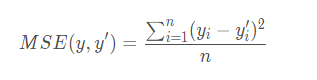Pyorch实现的MSE
import torch
import numpy as np

loss_fn = torch.nn.MSELoss(reduce=False, size_average=False)

a=np.array([[1,2],[3,4]])
b=np.array([[2,3],[4,5]])

loss = loss_fn(input.float(), target.float())
print(input.float())
print(target.float())

print(loss)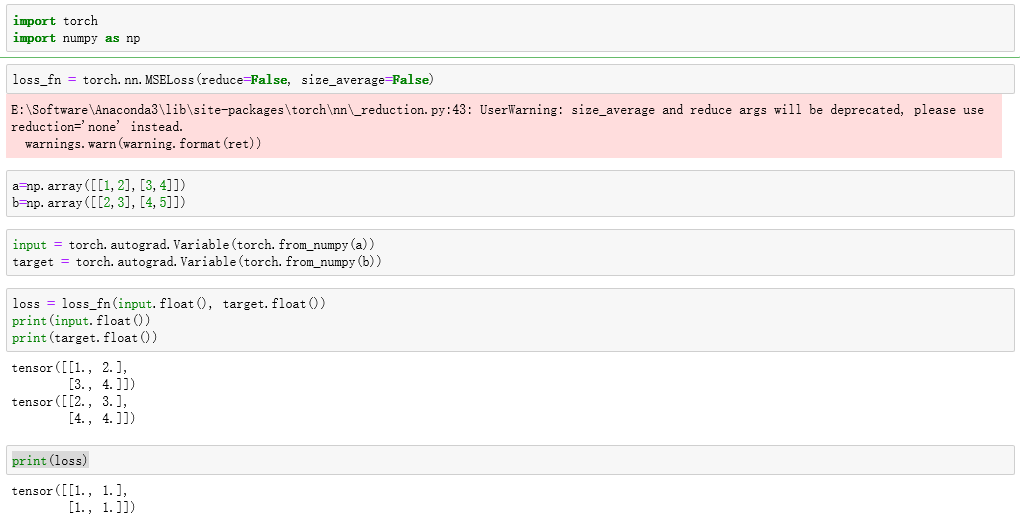a=np.array([[1,2],[3,4]])
b=np.array([[2,3],[4,6]])

loss_fn = torch.nn.MSELoss(reduce=True, size_average=True)

loss = loss_fn(input.float(), target.float())
print(input.float())
print(target.float())
print(loss)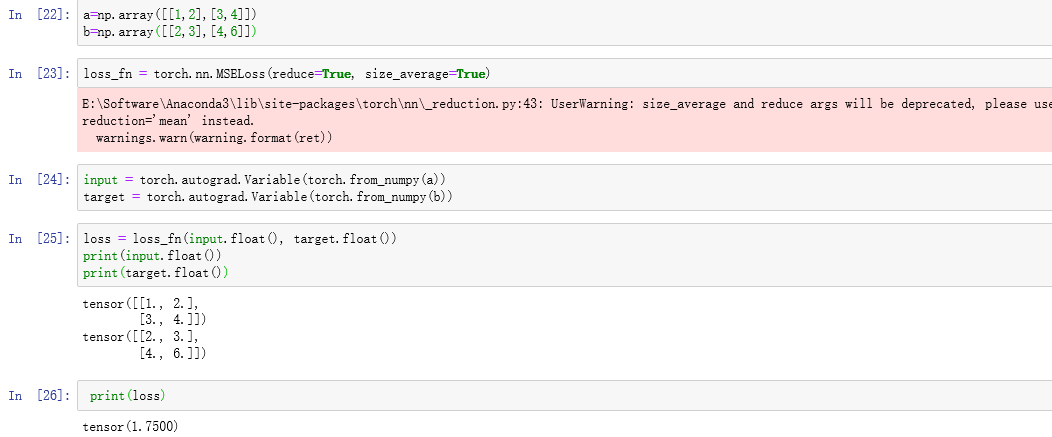展开全文• 比如对于一个4分类问题，期望输出应该为 g0=[0,1,0,0] ，实际输出为 g1=[0.2,0.4,0.4,0] ，计算g1与g0之间的差异所使用的方法，就是损失函数，分类问题中常用损失函数是交叉熵。 交叉熵（cross entropy）描述的是两...
交叉熵

分类问题中，预测结果是（或可以转化成）输入样本属于n个不同分类的对应概率。比如对于一个4分类问题，期望输出应该为 g0=[0,1,0,0] ，实际输出为 g1=[0.2,0.4,0.4,0] ，计算g1与g0之间的差异所使用的方法，就是损失函数，分类问题中常用损失函数是交叉熵。
交叉熵（cross entropy）描述的是两个概率分布之间的距离，距离越小表示这两个概率越相近，越大表示两个概率差异越大。对于两个概率分布 p 和 q ，使用 q 来表示 p 的交叉熵为：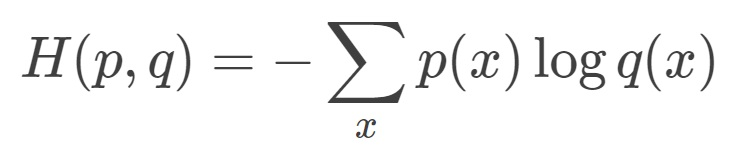由公式可以看出来，p 与 q 之间的交叉熵 和 q 与 p 之间的交叉熵不是等价的。上式表示的物理意义是使用概率分布 q 来表示概率分布 p 的困难程序，q 是预测值，p 是期望值。

神经网络的输出，也就是前向传播的输出可以通过Softmax回归变成概率分布，之后就可以使用交叉熵函数计算损失了。
交叉熵一般会跟Softmax一起使用，在tf中对这两个函数做了封装，就是 tf.nn.softmax_cross_entropy_with_logits 函数，可以直接计算神经网络的交叉熵损失。
cross_entropy = tf.nn.softmax_cross_entropy_with_logits(y, y_)

其中 y 是网络的输出，y_ 是期望输出。

针对分类任务中，正确答案往往只有一个的情况，tf提供了更加高效的 tf.nn.sparse_softmax_cross_entropy_with_logits 函数来求交叉熵损失。

均方误差

与分类任务对应的是回归问题，回归问题的任务是预测一个具体的数值，例如雨量预测、股价预测等。回归问题的网络输出一般只有一个节点，这个节点就是预测值。这种情况下就不方便使用交叉熵函数求损失函数了。
回归问题中常用的损失函数式均方误差(MSE,mean squared error)，定义如下：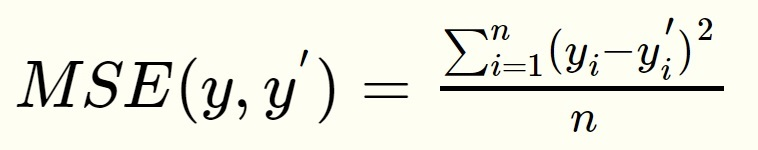均方误差的含义是求一个batch中n个样本的n个输出与期望输出的差的平方的平均值。
tf中实现均方误差的函数为：
mse = tf.reduce_mean(tf.square(y_ - y))

在有些特定场合，需要根据情况自定义损失函数，例如对于非常重要场所的安检工作，把一个正常物品错识别为危险品和把一个危险品错识别为正常品的损失显然是不一样的，宁可错判成危险品，不能漏判一个危险品，所以就要在定义损失函数的时候就要区别对待，对漏判加一个较大的比例系数。在tf中可以通过以下函数自定义：

loss = tf.reduce_sum(tf.select(tf.greater(v1,v2),loss1,loss2))

展开全文• 比如对于一个4分类问题，期望输出应该为g0=[0,1,0,0]，实际输出为g1=[0.2,0.4,0.4,0]，计算g1与g0之间的差异所使用的方法，就是损失函数，分类问题中常用损失函数是交叉熵。 交叉熵（cross entropy）描述的是两个...
交叉熵的对比二次方损失
https://blog.csdn.net/u012162613/article/details/44239919
这篇没看懂
https://blog.csdn.net/u014313009/article/details/51043064

分类问题中，预测结果是（或可以转化成）输入样本属于n个不同分类的对应概率。比如对于一个4分类问题，期望输出应该为 g0=[0,1,0,0] ，实际输出为 g1=[0.2,0.4,0.4,0] ，计算g1与g0之间的差异所使用的方法，就是损失函数，分类问题中常用损失函数是交叉熵。
交叉熵（cross entropy）描述的是两个概率分布之间的距离，距离越小表示这两个概率越相近，越大表示两个概率差异越大。对于两个概率分布 p 和 q ，使用 q 来表示 p 的交叉熵为：由公式可以看出来，p 与 q 之间的交叉熵 和 q 与 p 之间的交叉熵不是等价的。上式表示的物理意义是使用概率分布 q 来表示概率分布 p 的困难程序，q 是预测值，p 是期望值。

神经网络的输出，也就是前向传播的输出可以通过Softmax回归变成概率分布，之后就可以使用交叉熵函数计算损失了。
交叉熵一般会跟Softmax一起使用，在tf中对这两个函数做了封装，就是 tf.nn.softmax_cross_entropy_with_logits 函数，可以直接计算神经网络的交叉熵损失。
cross_entropy = tf.nn.softmax_cross_entropy_with_logits(y, y_)

其中 y 是网络的输出，y_ 是期望输出。

针对分类任务中，正确答案往往只有一个的情况，tf提供了更加高效的 tf.nn.sparse_softmax_cross_entropy_with_logits 函数来求交叉熵损失。

均方误差

与分类任务对应的是回归问题，回归问题的任务是预测一个具体的数值，例如雨量预测、股价预测等。回归问题的网络输出一般只有一个节点，这个节点就是预测值。这种情况下就不方便使用交叉熵函数求损失函数了。
回归问题中常用的损失函数式均方误差(MSE,mean squared error)，定义如下：均方误差的含义是求一个batch中n个样本的n个输出与期望输出的差的平方的平均值。
tf中实现均方误差的函数为：
mse = tf.reduce_mean(tf.square(y_ - y))

在有些特定场合，需要根据情况自定义损失函数，例如对于非常重要场所的安检工作，把一个正常物品错识别为危险品和把一个危险品错识别为正常品的损失显然是不一样的，宁可错判成危险品，不能漏判一个危险品，所以就要在定义损失函数的时候就要区别对待，对漏判加一个较大的比例系数。在tf中可以通过以下函数自定义：

loss = tf.reduce_sum(tf.select(tf.greater(v1,v2),loss1,loss2))

https://blog.csdn.net/dcrmg/article/details/80010342
展开全文• 本文将从代价函数的个人理解，均方误差代数函数作用原理以及在线性回归问题中为什么选择均方误差代数函数阐述。 1、代价函数的理解： 代价函数：真实的值与预测的值之间的偏差，由于偏差有可能正有可能负，因此使用...
在机器学习中，线性回归、逻辑回归问题总是绕不开代价函数。本文将从代价函数的个人理解，均方误差代数函数作用原理以及在线性回归问题中为什么选择均方误差代数函数阐述。
1、代价函数的理解：
代价函数：真实的值与预测的值之间的偏差，由于偏差有可能正有可能负，因此使用均方差来表示。代价函数的目的即用于找到最优解。
损失函数Loss Function：定义在单个样本上，算的是一个样本的误差。 代价函数Cost Function：定义在整个训练集上，算的是所有样本的误差，也就是损失函数的平均。 目标函数Object Function：定义为最终需要优化的函数。 Object Function = Cost Function（经验风险） + 正则化项（结构风险）
2、均方误差代数函数作用原理：
对于回归问题，代价函数常选择均方误差函数。下面阐述其作用原理： 对于假设函数：

h

θ

(

x

)

=

θ

0

+

θ

1

x

h_{\theta}(x)=\theta_{0}+\theta_{1} x

参数

θ

0

\theta_{0}

和

θ

1

\theta_{1}

两个参数的变化会导致假设函数的变化: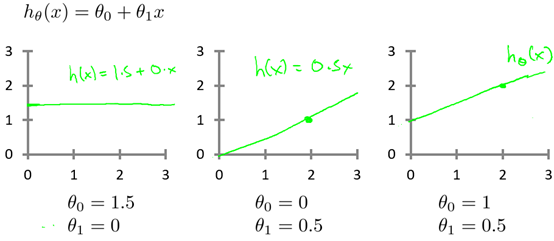实际的例子中，数据会以很多点的形式给出，回归问题就是将这些点拟合成一条曲线，即找到最优的参数

θ

0

\theta_{0}

和

θ

1

\theta_{1}

使得拟合的曲线更能代表所有的数据。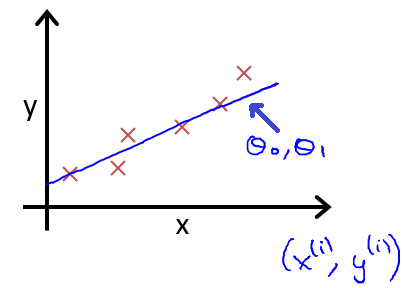如何找到最优参数

θ

0

\theta_{0}

和

θ

1

\theta_{1}

呢？使用代价函数！以均方误差代数函数为例： 从最简单的单一参数来看，假设函数为：

h

θ

(

x

)

=

θ

1

x

h_{\theta}(x)=\theta_{1} x

均方误差代数函数的主要思想就是将实际数据给出的值与拟合出的线的对应的值做差，求出拟合出的直线与实际的差距。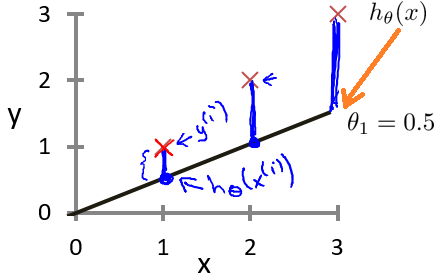为了使这个值不受个别极端数据的影响而产生巨大波动，采用类似方差再取二分之一的方式来减小个别数据影响。 这样，就产生了代价函数：

J

(

θ

0

,

θ

1

)

=

1

2

m

∑

i

=

1

m

(

h

θ

(

x

(

i

)

)

−

y

(

i

)

)

2

J\left(\theta_{0},\theta_{1}\right)=\frac{1}{2m}\sum_{i=1}^{m}\left(h_{\theta}\left(x^{(i)}\right)-y^{(i)}\right)^{2}

而最优解即为代价函数的最小值，根据以上公式多次计算可得到代价函数的图像：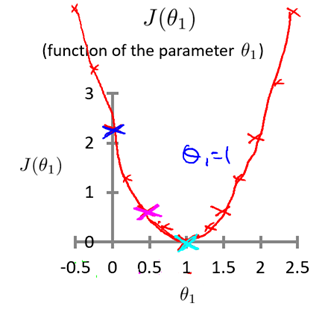可以轻易知道该代价函数的最小值(如何求解最小值？梯度下降算法~)，这时可以解出对应的 如果更多参数的话，图像就会更为复杂，两个参数的时候就已经是三维图像：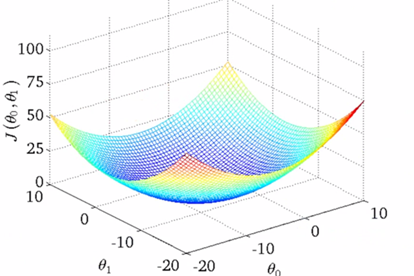高度即为均方误差代价函数的值，若参数再多则无法进行可视化，但其原理是相似的。 因此，我们可以终结得到：对于回归问题的代价函数，其最小值为：

minimize

⁡

θ

0

,

θ

1

J

(

θ

0

,

θ

1

)

\underset{\theta_{0}, \theta_{1}}{\operatorname{minimize}} J\left(\theta_{0}, \theta_{1}\right)

3、在回归问题中为什么选择均方误差函数：
从代价函数的理解考虑：代价是预测值和实际值之间的差距（两点之差），对于多个样本来说，就是差距之和。 将每一个样本的预测值和实际值之差相加，这不就是代价？但是注意到就每一个样本而言，代价有正有负，所有样本的代价加起来有可能出现正负抵消的情况，所以这并不是一个合适的代价函数（指将每一个样本的预测值和实际值之差相加）。 解决有正负的问题： 使用绝对值函数来表示代价，为了方便计算最小代价（计算最小代价可能用到最小二乘法），直接使用平方来衡量代价，即使用绝对值的平方来表示单个样本的代价，那么一个数据集的代价为：

(

h

θ

(

x

(

i

)

)

−

y

(

i

)

)

2

\left(h_{\theta}\left(x^{(i)}\right)-y^{(i)}\right)^{2}

即代价函数为：

J

(

θ

0

,

θ

1

)

=

1

2

m

∑

i

=

1

m

(

h

θ

(

x

(

i

)

)

−

y

(

i

)

)

2

J\left(\theta_{0},\theta_{1}\right)=\frac{1}{2m}\sum_{i=1}^{m}\left(h_{\theta}\left(x^{(i)}\right)-y^{(i)}\right)^{2}

为什么分母取2

m

m

而非

m

m

，是为了计算方便。
展开全文代价函数 线性回归
• ** 线性回归均方损失函数公式推导过程 ** 参考：https://www.cnblogs.com/ZhongliangXiang/p/8016635.html神经网络
• ## MSE（均方误差）函数和RMSE函数

万次阅读 多人点赞 2019-01-17 16:26:40MSE RMSE
• 有助于目录的生成如何改变文本的样式插入链接与图片如何插入一段漂亮的代码片生成一个适合你的列表创建一个表格设定内容居中、居左、居右...列表如何创建一个注脚注释也是必不可少的KaTeX数学公式新的甘特图功能...机器学习
• 数理统计中均方误差是指参数估计值与参数值之差平方的期望值，记为MSE。MSE是衡量“平均误差”的一种较方便的方法，MSE可以评价数据的变化程度，MSE的值越小，说明预测模型描述实验数据具有更好的精确度。SSE（和...
• 均方误差公式是E=½∑(yk-tk)²,yk代表神经网络的输出,tk代表监督数据,k表示数据的维数,神经网络的输出含义是经过了某种运算得出的最终结果,监督数据指的是正确的数据用来与最终输出相比较,维数的含义则是,得出的...python 人工智能 神经网络 数学
• 损失函数（loss function）可以为任意函数，常用的是均方误差和交叉熵误差 损失函数：最优参数解的参考指标，希望它越小越好 用于评价神经网络的性能“恶劣程度”，当前神经网络对监督数据（训练数据）在多大程度上...python
• 损失函数公式本身来说 1. 从损失函数公式的物理含义来说 MSE衡量的是预测值和目标值的欧式距离。 而交叉熵是一个信息论的概念，交叉熵能够衡量同一个随机变量中的两个不同概率分布的差异程度，在机器学习中就...
• 交叉熵（cross entropy）是常用的方法之一，刻画了两个概率分布之间的距离，是分类问题中使用较多的一种损失函数。 给定两个概率分布p和q，通过q来表示p的交叉熵为： 如何将神经网络前向传播得到的结果也变成...神经网络 MSE
• 函数形式 Sigmoid(x)=11+e−xSigmoid(x)=\frac{1}{1+e^{-x}}Sigmoid(x)=1+e−x1​ 函数图像 导数形式 Sigmoid′(x)=e−x(1+e−x)2=Sigmoid(x)(1−Sigmoid(x))Sigmoid'(x)=\frac{e^{-x}}{(1+e^{-x})^2}=Sigmoid(x)(1...神经网络 机器学习
• 转发：https://blog.csdn.net/xiongchengluo1129/article/details/79155550
• 与分类问题不同，回归问题解决的是对具体数值的预测，eg：房价预测，销量预测，流量预测等均方误差的定义：均方误差#y代表输出答案，y_代表标准答案 mse=tf.reduce_mean(tf.square(y_-y))但在实际问题中往往均方误差...
• 误差函数理解定义功能与BP算法，激活函数的关系误差函数的特点常见误差函数均方误差函数公式应用场景pytorch实现代码交叉熵公式应用场景pytorch实现代码 定义 误差函数是关于模型输出和样本标签值之差的函数，通过对...
• 我们设计神经网络的目的是为了实现一种模型，这个模型能够对数据做出合适的处理。比如分类问题，网络能够 以尽可能高的准确...首先，先说一下MSE，均方误差函数，这个函数在很多地方（常见于线性回归问题上，针对不...神经网络 算法
• MSE（均方误差）对于每一个输出的结果都非常看重，而交叉熵只对正确分类的结果看重。 例如：在一个三分类模型中，模型的输出结果为（a,b,c)，而真实的输出结果为(1,0,0)，那么MSE与cross-entropy相对应的损失函数的...
• 机器学习的讲义，主要介绍利用矩阵和最大似然估计推导最小均方误差函数
• mse(a) ----------------------------函数...MSE是（神经）网络的均方误差，叫"Mean Square Error"。比如有n对输入输出数据，每对为[Pi,Ti],i=1,2,...,n.网络通过训练后有网络输出,记为Yi。那MSE＝(求和(Ti-Yi)^2(i=1
• 计算均方误差MSE信噪比SNR峰值信噪比PSNR绝对平均误差的matlab函数 计算均方误差MSE信噪比SNR峰值信噪比PSNR绝对平均误差的matlab函数
• 没有约束条件，目标函数是若干二次项的和，每一项的形式如aTix−biaiTx−bia_i^Tx - b_i,具体形式如下： minimizef(x)=∑i=1k(aTix−bi)2minimizef(x)=∑i=1k(aiTx−bi)2 minimize \quad f(x) = \sum_{i=1}^{k}(a_...最小二乘法 最大似然 线性回归
• 翻译 | 张建军编辑 | 阿司匹林出品 | AI科技大本营机器学习中的所有算法都依赖于最小化或最大化某一个函数，我们称之为“目标函数”。最小化的这组函数被称为“损失函数”...
• 转者注：上面虽然使用的是Sigmoid函数，其实分类问题使用的是softmax函数，softmax可以看做sigmoid在对分类问题上的推广。 转者注：上面的y1′(1−y1′)y&#x27;_1(1-y&#x27;_1)y1′​(1−y1′​)是a对z...softmax
• 从上述的公式可以看出，交叉熵的损失函数只和分类正确的预测结果有关系，而MSE的损失函数还和错误的分类有关系，该分类函数除了让正确的分类尽量变大，还会让错误的分类变得平均，但实际在分类问题中这个调整是没有...分类
• 欧氏距离损失经常用在线性回归问题（求解的是连续问题）。 回归问题解决的是对具体数值的预测，比如房价预测、销量预测等等。 解决回归问题的神经网络一般只有一个输出节点，这个节点的输出值就是预测值。 二、数学......